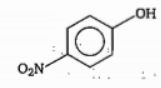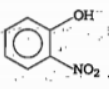Out of the two compounds shown below, the vapour pressure of B at a particular tempreture is expected to be :and(A)                                  (B)

(a) Highr than that of A

(b) Lower than that of A

(c) same as that of A

(d) can be higher or lower depending upon the size of the vessel

Concept Questions :-

Hydrogen Bonding
High Yielding Test Series + Question Bank - NEET 2020

Difficulty Level:

solubillity of alkali metal fluorides increases down the group select correct explanation for given statement :

(a) Hydration energy increase and lattice energy decrease down the group

(b) both energy decrease down the group but decrease in hyderation energy is rapid

(c) both energy decrease down the group but decrease in lattice energy is rapid

(d) both energy decrease down the group but increase in hyderation energy is rapid

Concept Questions :-

Lattice/Hydration Energy
High Yielding Test Series + Question Bank - NEET 2020

Difficulty Level:

In which of the following compounds B$—$F bond length is shortest ?

(a) $B{{F}_{4}}^{-}$       (b) $B{F}_{3}←N{H}_{3}$

(c) $B{F}_{3}$         (d) $B{F}_{3}←N{\left(C{H}_{3}\right)}_{3}$

Concept Questions :-

Hybridisation
High Yielding Test Series + Question Bank - NEET 2020

Difficulty Level:

In terms of polar character which of the following order is correct ?

(a) $N{H}_{3}<{H}_{2}O    (b) ${H}_{2}S

(c) ${H}_{2}O    (d) $HF<{H}_{2}O

Concept Questions :-

Polarity
High Yielding Test Series + Question Bank - NEET 2020

Difficulty Level:

The correct order or boiling point is:

(a) ${\mathrm{T}}_{2}<{\mathrm{D}}_{2}<{\mathrm{H}}_{2}$

(b) n-pentane<neo pentane

(c) Xe<Ar<He

(d) m-nitrophenol>o-nitrophenol

Concept Questions :-

Types of Bonding
High Yielding Test Series + Question Bank - NEET 2020

Difficulty Level:

The correct order of 'S$—$O' bond length is:

(a) $S{{O}_{3}}^{2-}>S{{O}_{4}}^{2-}>S{O}_{2}>S{O}_{2}$     (b) $S{{O}_{3}}^{2}>S{{O}^{2}}_{4}>S{O}_{2}>S{O}_{3}$

(c) $S{{O}_{4}}^{3}>S{{O}_{3}}^{2}>S{O}_{2}>S{O}_{3}$    (c) $S{{O}_{4}}^{2}>S{{O}_{3}}^{2}>S{O}_{3}>S{O}_{2}$

Concept Questions :-

M.O.T
High Yielding Test Series + Question Bank - NEET 2020

Difficulty Level:

The linear structure is possessed by :

(a) $SnC{l}_{2}$

(b) $NC{O}^{-}$

(c) $N{{O}_{2}}^{+}$

(d) $C{S}_{{}^{2}}$

Concept Questions :-

Hybridisation
High Yielding Test Series + Question Bank - NEET 2020

Difficulty Level:

Cis-butene dioic acid $\stackrel{{\mathrm{K}}_{{\mathrm{a}}_{1}}\left\{-{\mathrm{H}}^{+}\right\}}{⇌}{{\mathrm{X}}^{-}}_{1}\stackrel{{\mathrm{K}}_{{\mathrm{a}}_{2}}\left\{-{\mathrm{H}}^{+}\right\}}{⇌}{\mathrm{X}}_{2}^{2-}$

Trans-butene dioic acid $\stackrel{{\mathrm{K}}_{{\mathrm{a}}_{1}}\text{'}\left\{-{\mathrm{H}}^{+}\right\}}{⇌}{{\mathrm{Y}}^{-}}_{1}\stackrel{{\mathrm{K}}_{{\mathrm{a}}_{2}}·\left\{-{\mathrm{H}}^{+}\right\}}{⇌}{\mathrm{Y}}_{2}^{2-}$

The incorrect statement regarding above the information is:

(a) ${\mathrm{X}}_{2}^{2-}$ species is more basic than ${\mathrm{Y}}_{2}^{2-}$ species

(b) ${\mathrm{X}}_{1}^{-}$ species is more basic than ${\mathrm{Y}}_{1}^{-}$ species

(c) ${\mathrm{K}}_{\mathrm{a}1}$ is greater than $\mathrm{K}{\text{'}}_{\mathrm{a}2}$

(d) $\mathrm{K}{\text{'}}_{\mathrm{a}2}$ is greater than $\mathrm{K}{\text{'}}_{\mathrm{a}2}$

Concept Questions :-

Acid, Base and Fajan's Rule
High Yielding Test Series + Question Bank - NEET 2020

Difficulty Level:

In which of the following compounds octet is complete and incomplete for all atoms:

(a)                  IC               IC             IC                     C                         C

(b)                  C                IC             IC                     C                         IC

(c)                  C                IC             C                      IC                        IC

(d)                 IC                C              IC                    IC                        IC

where C denotes Complete and IC incomplete.

Concept Questions :-

Covalent Bonding
High Yielding Test Series + Question Bank - NEET 2020

Difficulty Level:

In which of the following metal of metal bond is present?

(a) Cupric chloride            (b) stannous chloride

(c) mercurous chloride      (d) mercuric chloride

Concept Questions :-

Types of Bonding